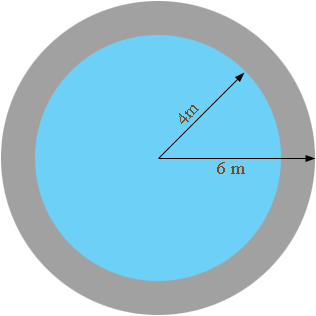SEARCH HOMEMath Central Quandaries & QueriesQuestion from adeniji, a teacher: find the area of a concrete path 2m wide surrounding a circular pond 12m in diameterThe area of a circle is π r2 square meters where r is the radius in meters. Find the area of a circle of radius 6 m and subtract the area of a circle of radius 4 m.

PennyMath Central is supported by the University of Regina and The Pacific Institute for the Mathematical Sciences.